### Friction: Equilibrium involving friction

If frictional forces act on a rigid body in equilibrium, the governing equations of friction should also be satisfied in addition to the equations of equilibrium. A stationary rigid body in equilibrium does not apparently slide against its surroundings. Therefore, the frictional forces acting on the body should either follow the non-slide, i.e.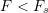, or impending slide, i.e.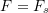, condition.

#### EXAMPLE 8.2.1

A person intends to push on a stationary chest of drawers on the floor as shown. If the weight of the unit is 300N and the coefficient of static friction is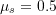, determine the magnitude of the pushing force that will cause the impending slide of the unit.

#### SOLUTION

Draw the FBD of the chest of drawers.

Note that the weight is applied at the geometric center (centroid) of the unit.

We need to check two cases: 1- impending slide, 2- tipping. The unit may tip before sliding, therefore, no sliding occurs at all. Note that only one of the two cases will happen.

1- Impending slide.

Assume that the unit is about to slide.

Therefore,

(1)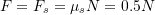.

Based on the FBD, we can write the equilibrium equations as,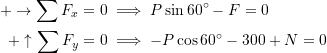Using Eq 1, the above equations become,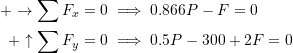forming a system of equations that leads to,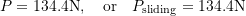2- Tipping

The unit may tip about its bottom right corner; meaning that it rotates about the corner. At the onset of tipping, the corner of the unit is is the only surface in contact with the floor, therefore,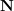acts at the corner, i.e.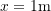. The FBD of the unit at the onset of tipping is then,

At the onset of tipping, the moment equilibrium must be satisfied, therefore,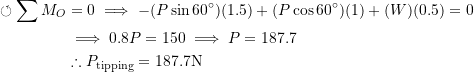Because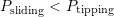, the unit will slide rather than tipping.

The magnitude of the pushing force that causes the unit to slide is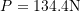.

#### EXAMPLE 8.2.2

A chest of drawers is at rest on a ramp with an inclination angle of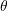. The coefficient of static friction between the unit and the ramp is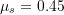. Determine whether the sliding or tipping happens first when increasing the inclination angle slowly.

#### SOLUTION

Draw the FBD of the unit.

Assume impending slide requiringand write the equations of equilibrium as,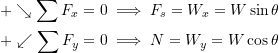With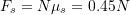, substituting this into the equilibrium equation for F_x and solving the system of two equations lead to,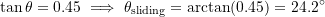We notice that the angle of repose,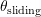, is equal to the angle of static friction.

To check for tipping, consider the onset of tipping at which the normal force acts at the corner of the unit as shown below.

At the onset of tipping the sum of moment about any point including the corner of the unit must be zero; write the moment equilibrium about the corner of the unit as,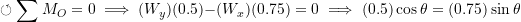Solving for, we get,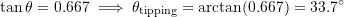Compareand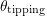with each other to determine the state of the motion of the unit.

Because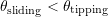, the unit will start sliding once the inclination angle reaches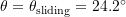.

Note that the magnitude of the weight of the unit was not needed to determine the slipping or tipping state of the box. The parameters directly affecting the state of the motion of the unit at a particular inclination angle are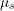(coefficient of static friction) for sliding, and the (ratio) of width to height of the unit for tipping.

Use the following interactive tool to investigate the forces (normal and friction) and the status of the motion of a box on an inclined plane. Note that the ratio of the dimensions (width and height) of the box affects the status of the motion.

#### EXAMPLE 8.2.3

Two blocks A and B, each having the same mass of 6kg, are connected by a linkage as shown. The structure is at rest. If the coefficients of static friction at the contact surfaces are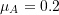and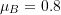, determine the largest magnitude of the vertical force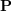that may be applied to pin C without causing the blocks to slide. The weights of the links are negligible and the pins of the links are frictionless. Assume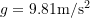.

#### SOLUTION

The direction of the frictional forces are determined based on the potential motion/slide of the boxes as shown.

To tackle this problem, the FBDs of the pin C, and the two blocks are separately considered for writing three sets of equations of equilibrium.

Pin C.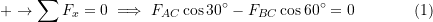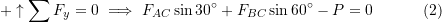Block A.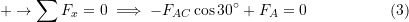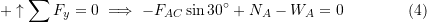Block B.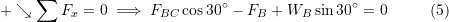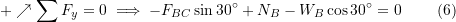Collecting all together, we have 6 equations,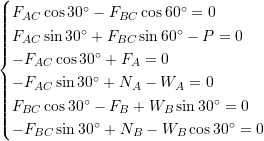and 7 unknowns,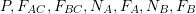.

Another equation, adding to the other six, comes from the condition on the friction. Assume that either block A or block B is about to slide, i.e. impending slide, and check the condition on the other block. Note that, it is not known which block(s) slides.

Case 1: Assume that block A is in impending slide.

In this case,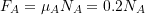. Therefore, the system of equations becomes,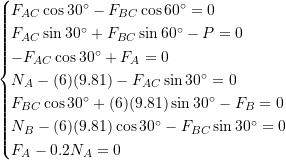Solving the system of equation by substitution, we get,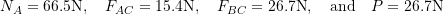Now, we need to check whether block B remains at rest or not.

Using Eqs. 5 and 6 obtained from the FBD of block B, we can write,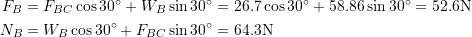The maximum value that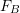can attain is,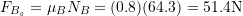Because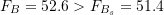, block B would slide before block A is at the onset of sliding (impending slide).

Case 2: Assume that block B is in impending slide.

In this case,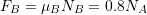. Therefore, the system of equations becomes,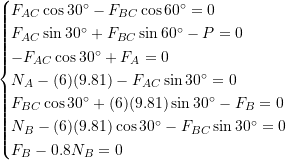Solving the system of equation by substitution, we get,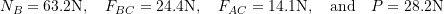Now, check the status of block A.

Using Eqs. 3 and 4 obtained from the FBD of block A, we can write,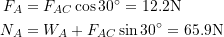The maximum value that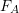can attain is,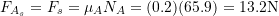Because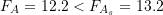, block A will not slide.

Consequently, the structure is in static equilibrium under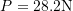with block B in impending slide and block A is not sliding.

Note: although we checked for the states of the motions of both blocks in each case, we just needed to calculate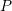in each case (either block A or block B in impending motion), without checking if the other block would slide under the calculated. The answer to the problem would be the smallestof both cases.

### Videos

Friction in Static Equilibrium:

Friction in Wheels, Wedges, and Multiple Objects: